# Gold Price Prediction Using Machine Learning In PythonIs it possible to predict where the Gold price is headed?

Yes, let’s use machine learning regression techniques to predict the price of one of the most important precious metal, the Gold.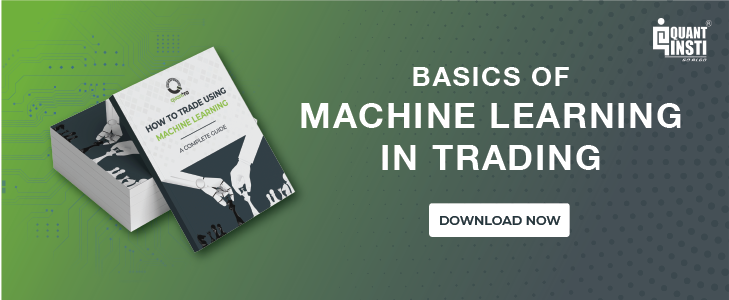We will create a machine learning linear regression model that takes information from the past Gold ETF (GLD) prices and returns a prediction of the Gold ETF price the next day.

GLD is the largest ETF to invest directly in physical gold. (source: http://www.etf.com/GLD)

### Steps to predict gold prices using machine learning in python

1. Import the libraries and read the Gold ETF data
2. Define explanatory variables
3. Define dependent variable
4. Split the data into train and test dataset
5. Create a linear regression model
6. Predict the Gold ETF prices

#### Import the libraries and read the Gold ETF data

First things first: import all the necessary libraries which are required to implement this strategy.

```# LinearRegression is a machine learning library for linear regression

from sklearn.linear_model import LinearRegression
# pandas and numpy are used for data manipulation

import pandas as pd

import numpy as np
# matplotlib and seaborn are used for plotting graphs

import matplotlib.pyplot as plt

import seaborn
# fix_yahoo_finance is used to fetch data

import fix_yahoo_finance as yf```

Then, we read the past 10 years of daily Gold ETF price data and store it in Df. We remove the columns which are not relevant and drop NaN values using dropna() function. Then, we plot the Gold ETF close price.

```# Read data

# Only keep close columns

Df=Df[['Close']]
# Drop rows with missing values

Df= Df.dropna()
# Plot the closing price of GLD

Df.Close.plot(figsize=(10,5))

plt.ylabel("Gold ETF Prices")

plt.show()```

Output: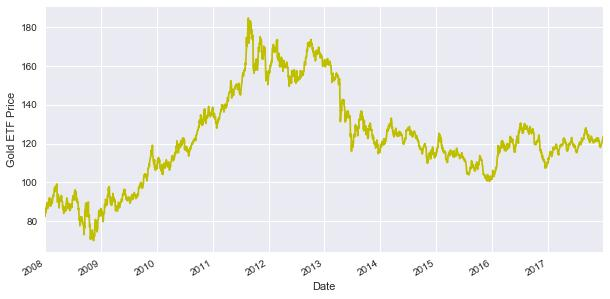#### Define explanatory variables

An explanatory variable is a variable that is manipulated to determine the value of the Gold ETF price the next day. Simply, they are the features which we want to use to predict the Gold ETF price. The explanatory variables in this strategy are the moving averages for past 3 days and 9 days. We drop the NaN values using dropna() function and store the feature variables in X.

However, you can add more variables to X which you think are useful to predict the prices of the Gold ETF. These variables can be technical indicators, the price of another ETF such as Gold miners ETF (GDX) or Oil ETF (USO), or US economic data.

```Df['S_3'] = Df['Close'].shift(1).rolling(window=3).mean()

Df['S_9']= Df['Close'].shift(1).rolling(window=9).mean()

Df= Df.dropna()

X = Df[['S_3','S_9']]

Output: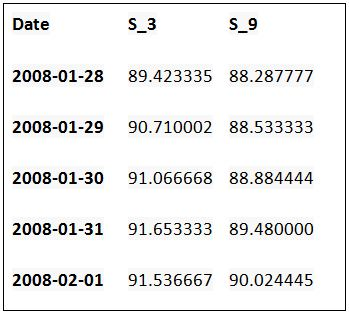#### Define dependent variable

Similarly, the dependent variable depends on the values of the explanatory variables. Simply put, it is the Gold ETF price which we are trying to predict. We store the Gold ETF price in y.

```y = Df['Close']

Output:

Date

2008-02-08    91.000000

2008-02-11    91.330002

2008-02-12    89.330002

2008-02-13    89.440002

2008-02-14    89.709999

Name: Close, dtype: float64

#### Split the data into train and test dataset

In this step, we split the predictors and output data into train and test data. The training data is used to create the linear regression model, by pairing the input with expected output. The test data is used to estimate how well the model has been trained.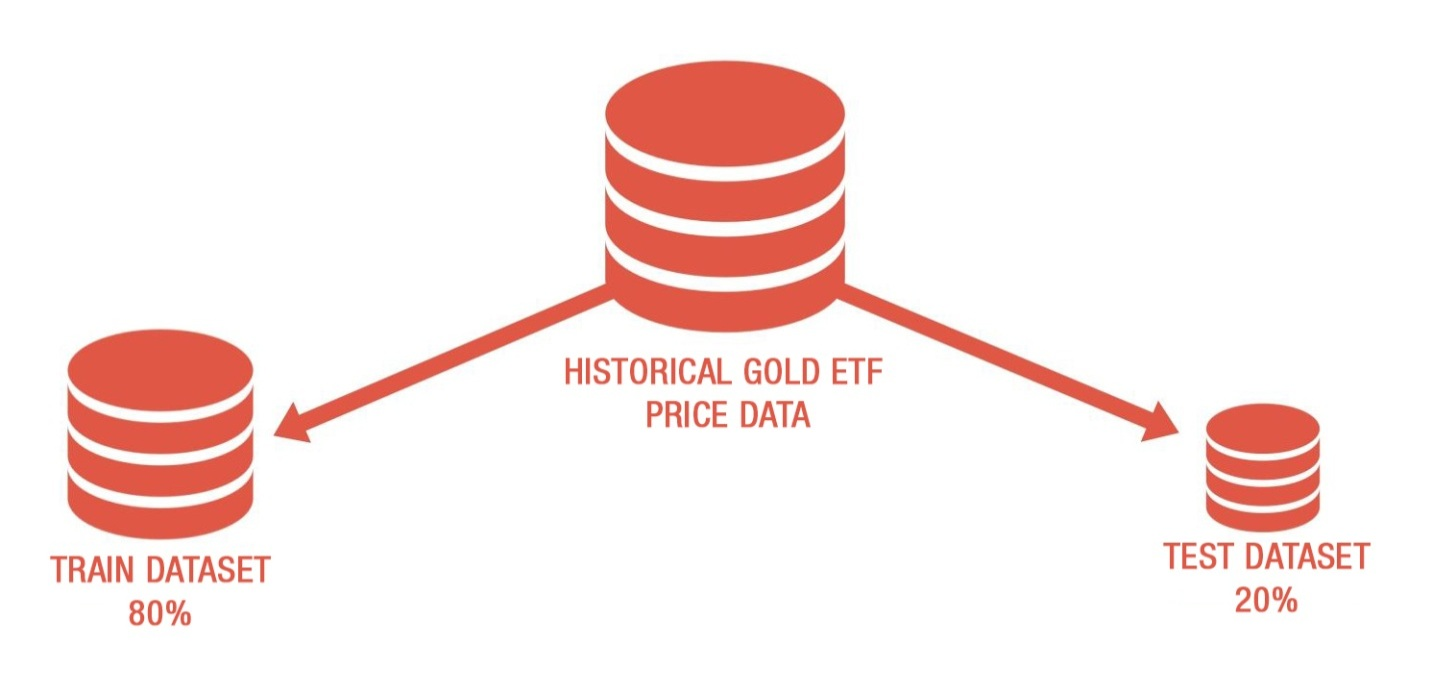1. First 80% of the data is used for training and remaining data for testing
2. X_train & y_train are training dataset
3. X_test & y_test are test dataset
```t=.8

t = int(t*len(Df))
# Train dataset

X_train = X[:t]

y_train = y[:t]
# Test dataset

X_test = X[t:]

y_test = y[t:]```

#### Create a linear regression model

We will now create a linear regression model. But, what is linear regression?

If we try to capture a mathematical relationship between ‘x’ and ‘y’ variables that “best” explains the observed values of ‘y’ in terms of observed values of ‘x’ by fitting a line through a scatter plots then such an equation between x and y is called linear regression analysis.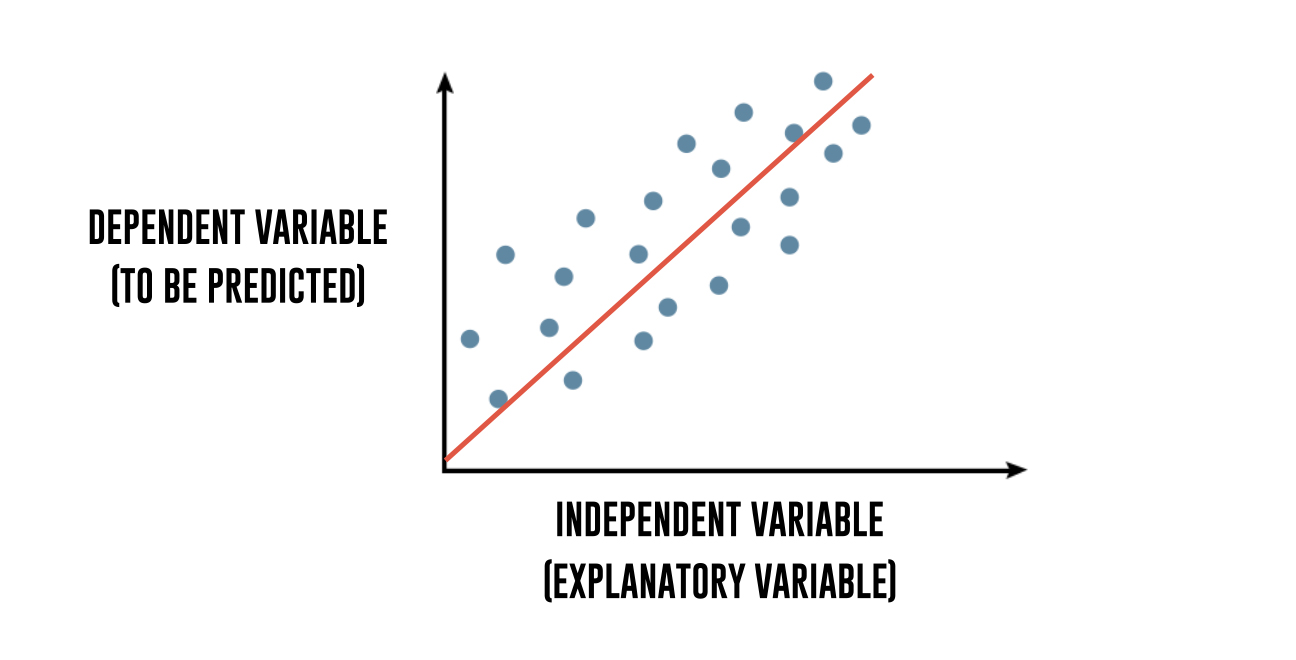To break it down further, regression explains the variation in a dependent variable in terms of independent variables. The dependent variable - ‘y’ is the variable that you want to predict. The independent variables - ‘x’ are the explanatory variables that you use to predict the dependent variable.  The following regression equation describes that relation:

```Y = m1 * X1 + m2 * X2 + C

Gold ETF price = m1 * 3 days moving average + m2 * 15 days moving average + c```

Then we use the fit method to fit the independent and dependent variables (x’s and y’s) to generate coefficient and constant for regression.

```linear = LinearRegression().fit(X_train,y_train)

print "Gold ETF Price =", round(linear.coef_,2), \

"* 3 Days Moving Average", round(linear.coef_,2), \

"* 9 Days Moving Average +", round(linear.intercept_,2)```

Output:

Gold ETF Price = 1.2 * 3 Days Moving Average - 0.2 * 9 Days Moving Average + 0.39

#### Predicting the Gold ETF prices

Now, it’s time to check if the model works in the test dataset. We predict the Gold ETF prices using the linear model created using the train dataset. The predict method finds the Gold ETF price (y) for the given explanatory variable X.

```predicted_price = linear.predict(X_test)

predicted_price = pd.DataFrame(predicted_price,index=y_test.index,columns = ['price'])

predicted_price.plot(figsize=(10,5))

y_test.plot()

plt.legend(['predicted_price','actual_price'])

plt.ylabel("Gold ETF Price")

plt.show()```

Output: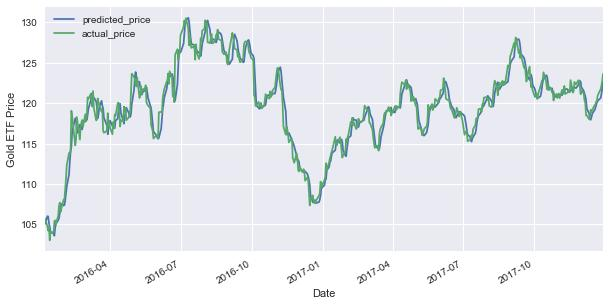The graph shows the predicted and actual price of the Gold ETF.

Now, let’s compute the goodness of the fit using the score() function.

```r2_score = linear.score(X[t:],y[t:])*100

float("{0:.2f}".format(r2_score))```

Output:

95.81%

As it can be seen, the R-squared of the model is 95.81%. R-squared is always between 0 and 100%. A score close to 100% indicates that the model explains the Gold ETF prices well.

Congrats! You just learned a fundamental yet strong machine learning technique. Thanks for reading!

### Next Step

Have an in-depth look into Pivot Point technique and using this in conjunction with candlestick charts for predicting market movement. Click here to read now.

Update

We have noticed that some users are facing challenges while downloading the market data from Yahoo and Google Finance platforms. In case you are looking for an alternative source for market data, you can use Quandl for the same.

Disclaimer: All investments and trading in the stock market involve risk. Any decisions to place trades in the financial markets, including trading in stock or options or other financial instruments is a personal decision that should only be made after thorough research, including a personal risk and financial assessment and the engagement of professional assistance to the extent you believe necessary. The trading strategies or related information mentioned in this article is for informational purposes only.

You can enroll for the online machine learning course on Quantra which covers classification algorithms, performance measures in machine learning, hyper-parameters, and building of supervised classifiers.

``````